# A Primer on Bayesian Multilevel Modeling using PyStan¶

Author: Chris Fonnesbeck
License: Apache, Version 2.0 (code); CC-BY 3.0 (text)

Hierarchical or multilevel modeling is a generalization of regression modeling.

Multilevel models are regression models in which the constituent model parameters are given probability models. This implies that model parameters are allowed to vary by group.

Observational units are often naturally clustered. Clustering induces dependence between observations, despite random sampling of clusters and random sampling within clusters.

A hierarchical model is a particular multilevel model where parameters are nested within one another.

Some multilevel structures are not hierarchical.

• e.g. "country" and "year" are not nested, but may represent separate, but overlapping, clusters of parameters

We will motivate this topic using an environmental epidemiology example.

### Example: Radon contamination (Gelman and Hill 2006)¶

Radon is a radioactive gas that enters homes through contact points with the ground. It is a carcinogen that is the primary cause of lung cancer in non-smokers. Radon levels vary greatly from household to household.The EPA did a study of radon levels in 80,000 houses. Two important predictors:

• measurement in basement or first floor (radon higher in basements)
• county uranium level (positive correlation with radon levels)

We will focus on modeling radon levels in Minnesota.

The hierarchy in this example is households within county.

### Data organization¶

First, we import the data from a local file, and extract Minnesota's data.

In :
%matplotlib inline
import numpy as np
import pandas as pd
import matplotlib.pyplot as plt
import seaborn as sns; sns.set_context('notebook')

srrs2.columns = srrs2.columns.map(str.strip)
srrs_mn = srrs2.assign(fips=srrs2.stfips*1000 + srrs2.cntyfips)[srrs2.state=='MN']


Next, obtain the county-level predictor, uranium, by combining two variables.

In :
cty = pd.read_csv('../data/cty.dat')
cty_mn = cty[cty.st=='MN'].copy()
cty_mn[ 'fips'] = 1000*cty_mn.stfips + cty_mn.ctfips


Use the merge method to combine home- and county-level information in a single DataFrame.

In :
srrs_mn = srrs_mn.merge(cty_mn[['fips', 'Uppm']], on='fips')
srrs_mn = srrs_mn.drop_duplicates(subset='idnum')
u = np.log(srrs_mn.Uppm)

n = len(srrs_mn)

In :
srrs_mn.head()

Out:
idnum state state2 stfips zip region typebldg floor room basement ... stopdt activity pcterr adjwt dupflag zipflag cntyfips county fips Uppm
0 5081 MN MN 27 55735 5 1 1 3 N ... 12288 2.2 9.7 1146.499190 1 0 1 AITKIN 27001 0.502054
1 5082 MN MN 27 55748 5 1 0 4 Y ... 12088 2.2 14.5 471.366223 0 0 1 AITKIN 27001 0.502054
2 5083 MN MN 27 55748 5 1 0 4 Y ... 21188 2.9 9.6 433.316718 0 0 1 AITKIN 27001 0.502054
3 5084 MN MN 27 56469 5 1 0 4 Y ... 123187 1.0 24.3 461.623670 0 0 1 AITKIN 27001 0.502054
4 5085 MN MN 27 55011 3 1 0 4 Y ... 13088 3.1 13.8 433.316718 0 0 3 ANOKA 27003 0.428565

5 rows × 27 columns

We also need a lookup table (dict) for each unique county, for indexing.

In :
srrs_mn.county = srrs_mn.county.str.strip()
mn_counties = srrs_mn.county.unique()
counties = len(mn_counties)


Finally, create local copies of variables.

In :
county_lookup = dict(zip(mn_counties, range(len(mn_counties))))
county = srrs_mn['county_code'] = srrs_mn.county.replace(county_lookup).values
floor_measure = srrs_mn.floor.values


Distribution of radon levels in MN (log scale):

In :
srrs_mn.activity.apply(lambda x: np.log(x+0.1)).hist(bins=25)

Out:
<matplotlib.axes._subplots.AxesSubplot at 0x114b64940>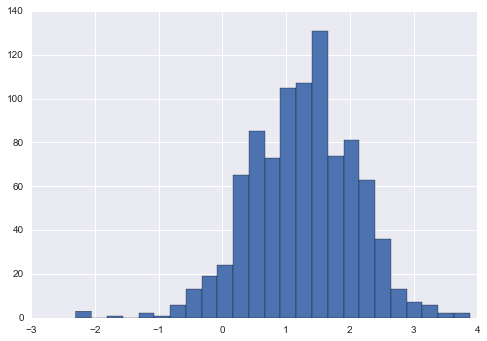## Conventional approaches¶

The two conventional alternatives to modeling radon exposure represent the two extremes of the bias-variance tradeoff:

Complete pooling:

Treat all counties the same, and estimate a single radon level.

$$y_i = \alpha + \beta x_i + \epsilon_i$$

No pooling:

Model radon in each county independently.

$$y_i = \alpha_{j[i]} + \beta x_i + \epsilon_i$$

where $j = 1,\ldots,85$

The errors $\epsilon_i$ may represent measurement error, temporal within-house variation, or variation among houses.

To specify this model in Stan, we begin by constructing the data block, which includes vectors of log-radon measurements (y) and floor measurement covariates (x), as well as the number of samples (N).

In :
pooled_data = """
data {
int<lower=0> N;
vector[N] x;
vector[N] y;
}
"""


Next we initialize our parameters, which in this case are the linear model coefficients and the normal scale parameter. Notice that sigma is constrained to be positive.

In :
pooled_parameters = """
parameters {
vector beta;
real<lower=0> sigma;
}
"""


Finally, we model the log-radon measurements as a normal sample with a mean that is a function of the floor measurement.

In :
pooled_model = """
model {
y ~ normal(beta + beta * x, sigma);
}
"""


We then pass the code, data, and parameters to the stan function. The sampling requires specifying how many iterations we want, and how many parallel chains to sample. Here, we will sample 2 chains of length 1000.

In :
import pystan

'x': floor_measure,

pooled_fit = pystan.stan(model_code=pooled_data + pooled_parameters + pooled_model,
data=pooled_data_dict, iter=1000, chains=2)


The sample can be extracted for plotting and summarization.

In :
pooled_sample = pooled_fit.extract(permuted=True)

In :
b0, m0 = pooled_sample['beta'].T.mean(1)

In :
plt.scatter(srrs_mn.floor, np.log(srrs_mn.activity+0.1))
xvals = np.linspace(-0.2, 1.2)
plt.plot(xvals, m0*xvals+b0, 'r--')

Out:
[<matplotlib.lines.Line2D at 0x119b13fd0>]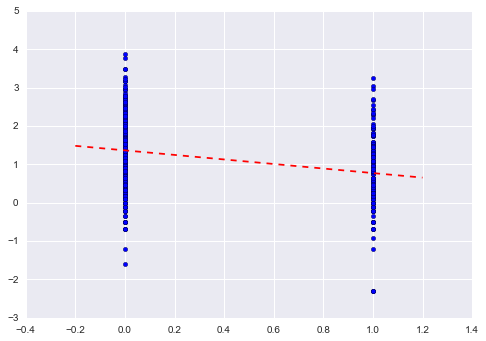At the other end of the extreme, we can fit separate (independent) means for each county. The only things that are shared in this model are the coefficient for the basement measurement effect, and the standard deviation of the error.

In :
unpooled_model = """data {
int<lower=0> N;
int<lower=1,upper=85> county[N];
vector[N] x;
vector[N] y;
}
parameters {
vector a;
real beta;
real<lower=0,upper=100> sigma;
}
transformed parameters {
vector[N] y_hat;

for (i in 1:N)
y_hat[i] <- beta * x[i] + a[county[i]];
}
model {
y ~ normal(y_hat, sigma);
}"""

In :
unpooled_data = {'N': len(log_radon),
'county': county+1, # Stan counts starting at 1
'x': floor_measure,

unpooled_fit = pystan.stan(model_code=unpooled_model, data=unpooled_data, iter=1000, chains=2)

In :
unpooled_estimates = pd.Series(unpooled_fit['a'].mean(0), index=mn_counties)
unpooled_se = pd.Series(unpooled_fit['a'].std(0), index=mn_counties)


We can plot the ordered estimates to identify counties with high radon levels:

In :
order = unpooled_estimates.sort_values().index

plt.scatter(range(len(unpooled_estimates)), unpooled_estimates[order])
for i, m, se in zip(range(len(unpooled_estimates)), unpooled_estimates[order], unpooled_se[order]):
plt.plot([i,i], [m-se, m+se], 'b-')
plt.xlim(-1,86); plt.ylim(-1,4)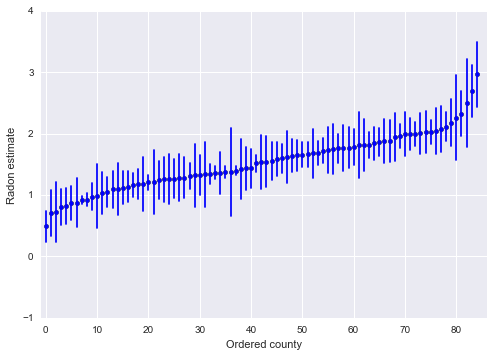Here are visual comparisons between the pooled and unpooled estimates for a subset of counties representing a range of sample sizes.

In :
sample_counties = ('LAC QUI PARLE', 'AITKIN', 'KOOCHICHING',
'DOUGLAS', 'CLAY', 'STEARNS', 'RAMSEY', 'ST LOUIS')

fig, axes = plt.subplots(2, 4, figsize=(12, 6), sharey=True, sharex=True)
axes = axes.ravel()
m = unpooled_fit['beta'].mean(0)
for i,c in enumerate(sample_counties):
x = srrs_mn.floor[srrs_mn.county==c]
axes[i].scatter(x + np.random.randn(len(x))*0.01, y, alpha=0.4)

# No pooling model
b = unpooled_estimates[c]

# Plot both models and data
xvals = np.linspace(-0.2, 1.2)
axes[i].plot(xvals, m*xvals+b)
axes[i].plot(xvals, m0*xvals+b0, 'r--')
axes[i].set_xticks([0,1])
axes[i].set_xticklabels(['basement', 'floor'])
axes[i].set_ylim(-1, 3)
axes[i].set_title(c)
if not i%2: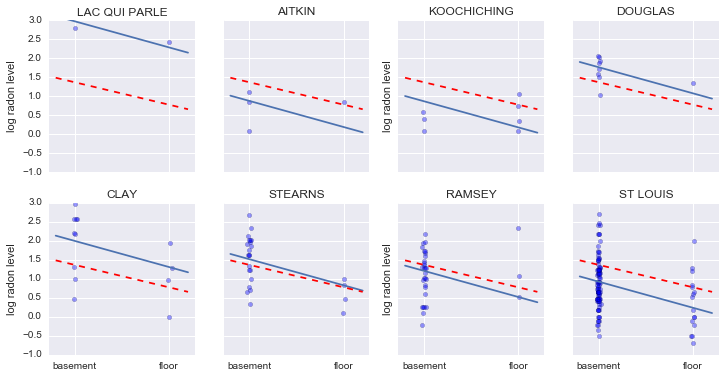Neither of these models are satisfactory:

• if we are trying to identify high-radon counties, pooling is useless
• we do not trust extreme unpooled estimates produced by models using few observations

## Multilevel and hierarchical models¶

When we pool our data, we imply that they are sampled from the same model. This ignores any variation among sampling units (other than sampling variance):When we analyze data unpooled, we imply that they are sampled independently from separate models. At the opposite extreme from the pooled case, this approach claims that differences between sampling units are to large to combine them:In a hierarchical model, parameters are viewed as a sample from a population distribution of parameters. Thus, we view them as being neither entirely different or exactly the same. This is parital pooling.We can use PyStan to easily specify multilevel models, and fit them using Hamiltonian Monte Carlo.

## Partial pooling model¶

The simplest partial pooling model for the household radon dataset is one which simply estimates radon levels, without any predictors at any level. A partial pooling model represents a compromise between the pooled and unpooled extremes, approximately a weighted average (based on sample size) of the unpooled county estimates and the pooled estimates.

$$\hat{\alpha} \approx \frac{(n_j/\sigma_y^2)\bar{y}_j + (1/\sigma_{\alpha}^2)\bar{y}}{(n_j/\sigma_y^2) + (1/\sigma_{\alpha}^2)}$$
• Estimates for counties with smaller sample sizes will shrink towards the state-wide average.
• Estimates for counties with larger sample sizes will be closer to the unpooled county estimates.
In :
partial_pooling = """
data {
int<lower=0> N;
int<lower=1,upper=85> county[N];
vector[N] y;
}
parameters {
vector a;
real mu_a;
real<lower=0,upper=100> sigma_a;
real<lower=0,upper=100> sigma_y;
}
transformed parameters {
vector[N] y_hat;
for (i in 1:N)
y_hat[i] <- a[county[i]];
}
model {
mu_a ~ normal(0, 1);
a ~ normal (10 * mu_a, sigma_a);

y ~ normal(y_hat, sigma_y);
}"""


Notice now we have two standard deviations, one describing the residual error of the observations, and another the variability of the county means around the average.

In :
partial_pool_data = {'N': len(log_radon),
'county': county+1, # Stan counts starting at 1

partial_pool_fit = pystan.stan(model_code=partial_pooling, data=partial_pool_data, iter=1000, chains=2)

In :
sample_trace = partial_pool_fit['a']

fig, axes = plt.subplots(1, 2, figsize=(14,6), sharex=True, sharey=True)
samples, counties = sample_trace.shape
jitter = np.random.normal(scale=0.1, size=counties)

n_county = srrs_mn.groupby('county')['idnum'].count()
unpooled = pd.DataFrame({'n':n_county, 'm':unpooled_means, 'sd':unpooled_sd})
unpooled['se'] = unpooled.sd/np.sqrt(unpooled.n)

axes.plot(unpooled.n + jitter, unpooled.m, 'b.')
for j, row in zip(jitter, unpooled.iterrows()):
name, dat = row
axes.plot([dat.n+j,dat.n+j], [dat.m-dat.se, dat.m+dat.se], 'b-')
axes.set_xscale('log')
axes.hlines(sample_trace.mean(), 0.9, 100, linestyles='--')

samples, counties = sample_trace.shape
means = sample_trace.mean(axis=0)
sd = sample_trace.std(axis=0)
axes.scatter(n_county.values + jitter, means)
axes.set_xscale('log')
axes.set_xlim(1,100)
axes.set_ylim(0, 3)
axes.hlines(sample_trace.mean(), 0.9, 100, linestyles='--')
for j,n,m,s in zip(jitter, n_county.values, means, sd):
axes.plot([n+j]*2, [m-s, m+s], 'b-')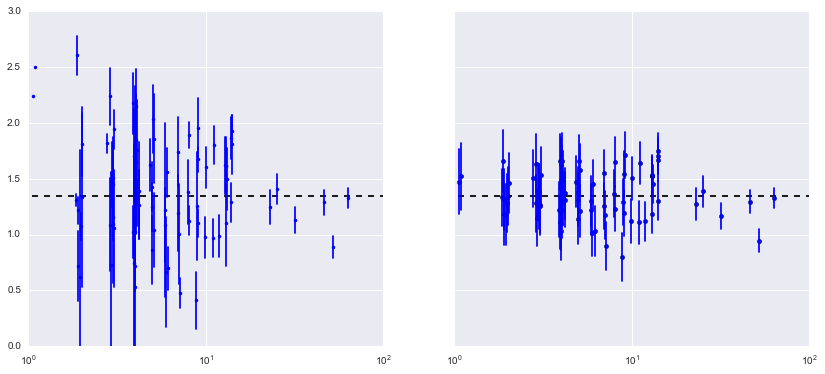Notice the difference between the unpooled and partially-pooled estimates, particularly at smaller sample sizes. The former are both more extreme and more imprecise.

## Varying intercept model¶

This model allows intercepts to vary across county, according to a random effect.

$$y_i = \alpha_{j[i]} + \beta x_{i} + \epsilon_i$$

where

$$\epsilon_i \sim N(0, \sigma_y^2)$$

and the intercept random effect:

$$\alpha_{j[i]} \sim N(\mu_{\alpha}, \sigma_{\alpha}^2)$$

As with the the “no-pooling” model, we set a separate intercept for each county, but rather than fitting separate least squares regression models for each county, multilevel modeling shares strength among counties, allowing for more reasonable inference in counties with little data.

In :
varying_intercept = """
data {
int<lower=0> J;
int<lower=0> N;
int<lower=1,upper=J> county[N];
vector[N] x;
vector[N] y;
}
parameters {
vector[J] a;
real b;
real mu_a;
real<lower=0,upper=100> sigma_a;
real<lower=0,upper=100> sigma_y;
}
transformed parameters {

vector[N] y_hat;

for (i in 1:N)
y_hat[i] <- a[county[i]] + x[i] * b;
}
model {
sigma_a ~ uniform(0, 100);
a ~ normal (mu_a, sigma_a);

b ~ normal (0, 1);

sigma_y ~ uniform(0, 100);
y ~ normal(y_hat, sigma_y);
}
"""

In :
varying_intercept_data = {'N': len(log_radon),
'J': len(n_county),
'county': county+1, # Stan counts starting at 1
'x': floor_measure,

varying_intercept_fit = pystan.stan(model_code=varying_intercept, data=varying_intercept_data, iter=1000, chains=2)

In :
a_sample = pd.DataFrame(varying_intercept_fit['a'])

In :
import seaborn as sns
sns.set(style="ticks", palette="muted", color_codes=True)

# Plot the orbital period with horizontal boxes
plt.figure(figsize=(16, 6))
sns.boxplot(data=a_sample, whis=np.inf, color="c")

Out:
<matplotlib.axes._subplots.AxesSubplot at 0x118f5fb70>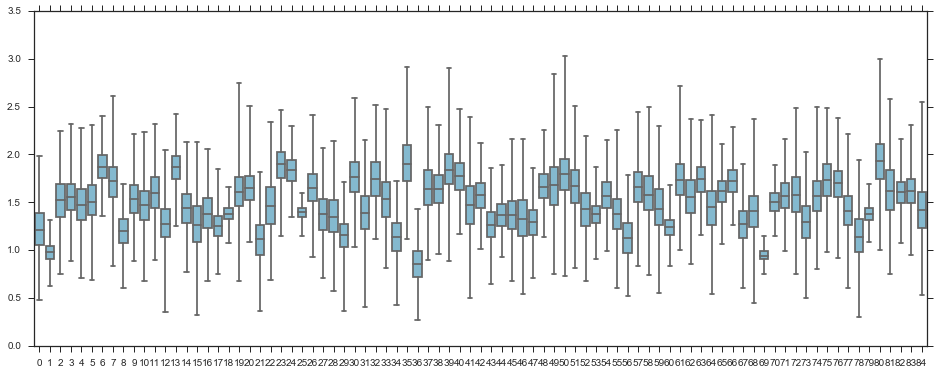In :
varying_intercept_fit.plot(pars=['sigma_a', 'b']);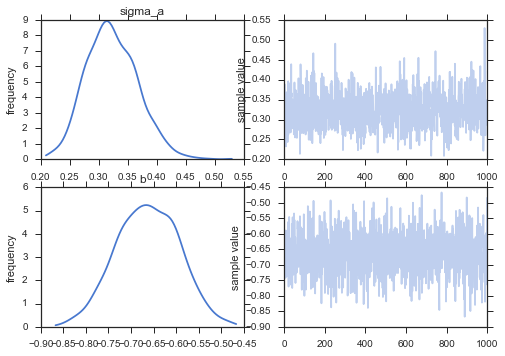The estimate for the floor coefficient is approximately -0.66, which can be interpreted as houses without basements having about half ($\exp(-0.66) = 0.52$) the radon levels of those with basements, after accounting for county.

In :
varying_intercept_fit['b'].mean()

Out:
-0.66292666591258154
In :
xvals = np.arange(2)
bp = varying_intercept_fit['a'].mean(axis=0)
mp = varying_intercept_fit['b'].mean()
for bi in bp:
plt.plot(xvals, mp*xvals + bi, 'bo-', alpha=0.4)
plt.xlim(-0.1,1.1);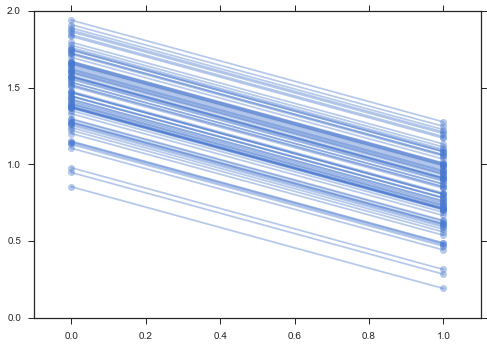It is easy to show that the partial pooling model provides more objectively reasonable estimates than either the pooled or unpooled models, at least for counties with small sample sizes.

In :
fig, axes = plt.subplots(2, 4, figsize=(12, 6), sharey=True, sharex=True)
axes = axes.ravel()
for i,c in enumerate(sample_counties):

# Plot county data
x = srrs_mn.floor[srrs_mn.county==c]
axes[i].scatter(x + np.random.randn(len(x))*0.01, y, alpha=0.4)

# No pooling model
m,b = unpooled_estimates[['floor', c]]

xvals = np.linspace(-0.2, 1.2)
# Unpooled estimate
axes[i].plot(xvals, m*xvals+b)
# Pooled estimate
axes[i].plot(xvals, m0*xvals+b0, 'r--')
# Partial pooling esimate
axes[i].plot(xvals, mp*xvals+bp[county_lookup[c]], 'k:')
axes[i].set_xticks([0,1])
axes[i].set_xticklabels(['basement', 'floor'])
axes[i].set_ylim(-1, 3)
axes[i].set_title(c)
if not i%2: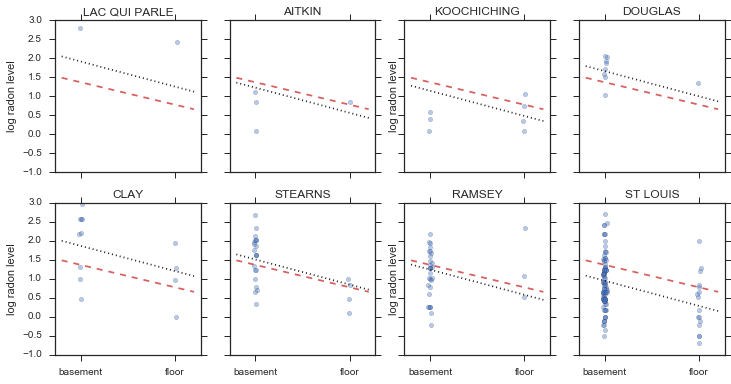## Varying slope model¶

Alternatively, we can posit a model that allows the counties to vary according to how the location of measurement (basement or floor) influences the radon reading.

$$y_i = \alpha + \beta_{j[i]} x_{i} + \epsilon_i$$
In :
varying_slope = """
data {
int<lower=0> J;
int<lower=0> N;
int<lower=1,upper=J> county[N];
vector[N] x;
vector[N] y;
}
parameters {
real a;
vector[J] b;
real mu_b;
real<lower=0,upper=100> sigma_b;
real<lower=0,upper=100> sigma_y;
}
transformed parameters {

vector[N] y_hat;

for (i in 1:N)
y_hat[i] <- a + x[i] * b[county[i]];
}
model {
sigma_b ~ uniform(0, 100);
b ~ normal (mu_b, sigma_b);

a ~ normal (0, 1);

sigma_y ~ uniform(0, 100);
y ~ normal(y_hat, sigma_y);
}
"""

In :
varying_slope_data = {'N': len(log_radon),
'J': len(n_county),
'county': county+1, # Stan counts starting at 1
'x': floor_measure,

varying_slope_fit = pystan.stan(model_code=varying_slope, data=varying_slope_data, iter=1000, chains=2)

In :
b_sample = pd.DataFrame(varying_slope_fit['b'])

In :
# Plot the orbital period with horizontal boxes
plt.figure(figsize=(16, 6))
sns.boxplot(data=b_sample, whis=np.inf, color="c")

Out:
<matplotlib.axes._subplots.AxesSubplot at 0x119368f60>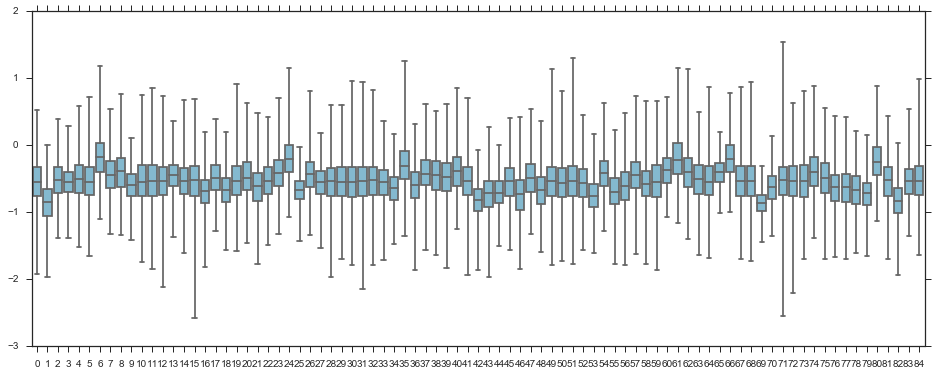In :
xvals = np.arange(2)
b = varying_slope_fit['a'].mean()
m = varying_slope_fit['b'].mean(axis=0)
for mi in m:
plt.plot(xvals, mi*xvals + b, 'bo-', alpha=0.4)
plt.xlim(-0.2, 1.2);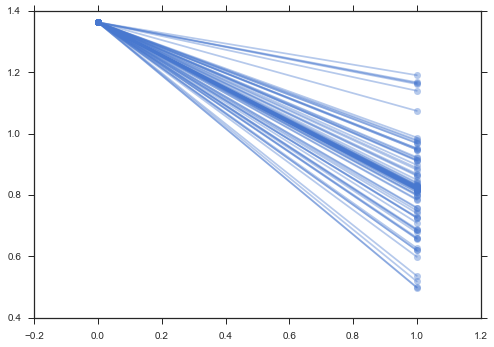## Varying intercept and slope model¶

The most general model allows both the intercept and slope to vary by county:

$$y_i = \alpha_{j[i]} + \beta_{j[i]} x_{i} + \epsilon_i$$
In :
varying_intercept_slope = """
data {
int<lower=0> N;
int<lower=0> J;
vector[N] y;
vector[N] x;
int county[N];
}
parameters {
real<lower=0> sigma;
real<lower=0> sigma_a;
real<lower=0> sigma_b;
vector[J] a;
vector[J] b;
real mu_a;
real mu_b;
}

model {
mu_a ~ normal(0, 100);
mu_b ~ normal(0, 100);

a ~ normal(mu_a, sigma_a);
b ~ normal(mu_b, sigma_b);
y ~ normal(a[county] + b[county].*x, sigma);
}
"""

In :
varying_intercept_slope_data = {'N': len(log_radon),
'J': len(n_county),
'county': county+1, # Stan counts starting at 1
'x': floor_measure,

varying_intercept_slope_fit = pystan.stan(model_code=varying_intercept_slope,
data=varying_intercept_slope_data,
iter=1000, chains=2)

In :
xvals = np.arange(2)
b = varying_intercept_slope_fit['a'].mean(axis=0)
m = varying_intercept_slope_fit['b'].mean(axis=0)
for bi,mi in zip(b,m):
plt.plot(xvals, mi*xvals + bi, 'bo-', alpha=0.4)
plt.xlim(-0.1, 1.1);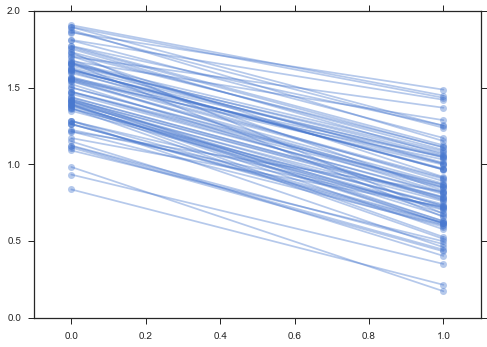A primary strength of multilevel models is the ability to handle predictors on multiple levels simultaneously. If we consider the varying-intercepts model above:

$$y_i = \alpha_{j[i]} + \beta x_{i} + \epsilon_i$$

we may, instead of a simple random effect to describe variation in the expected radon value, specify another regression model with a county-level covariate. Here, we use the county uranium reading $u_j$, which is thought to be related to radon levels:

$$\alpha_j = \gamma_0 + \gamma_1 u_j + \zeta_j$$$$\zeta_j \sim N(0, \sigma_{\alpha}^2)$$

Thus, we are now incorporating a house-level predictor (floor or basement) as well as a county-level predictor (uranium).

Note that the model has both indicator variables for each county, plus a county-level covariate. In classical regression, this would result in collinearity. In a multilevel model, the partial pooling of the intercepts towards the expected value of the group-level linear model avoids this.

Group-level predictors also serve to reduce group-level variation $\sigma_{\alpha}$. An important implication of this is that the group-level estimate induces stronger pooling.

In :
hierarchical_intercept = """
data {
int<lower=0> J;
int<lower=0> N;
int<lower=1,upper=J> county[N];
vector[N] u;
vector[N] x;
vector[N] y;
}
parameters {
vector[J] a;
vector b;
real mu_a;
real<lower=0,upper=100> sigma_a;
real<lower=0,upper=100> sigma_y;
}
transformed parameters {
vector[N] y_hat;
vector[N] m;

for (i in 1:N) {
m[i] <- a[county[i]] + u[i] * b;
y_hat[i] <- m[i] + x[i] * b;
}
}
model {
mu_a ~ normal(0, 1);
a ~ normal(mu_a, sigma_a);
b ~ normal(0, 1);
y ~ normal(y_hat, sigma_y);
}
"""

In :
hierarchical_intercept_data = {'N': len(log_radon),
'J': len(n_county),
'county': county+1, # Stan counts starting at 1
'u': u,
'x': floor_measure,

hierarchical_intercept_fit = pystan.stan(model_code=hierarchical_intercept, data=hierarchical_intercept_data,
iter=1000, chains=2)

In :
#a_means = M_hierarchical.a.trace().mean(axis=0)
m_means = hierarchical_intercept_fit['m'].mean(axis=0)
plt.scatter(u, m_means)
g0 = hierarchical_intercept_fit['mu_a'].mean()
g1 = hierarchical_intercept_fit['b'][:, 0].mean()
xvals = np.linspace(-1, 0.8)
plt.plot(xvals, g0+g1*xvals, 'k--')
plt.xlim(-1, 0.8)

m_se = hierarchical_intercept_fit['m'].std(axis=0)
for ui, m, se in zip(u, m_means, m_se):
plt.plot([ui,ui], [m-se, m+se], 'b-')
plt.xlabel('County-level uranium'); plt.ylabel('Intercept estimate')

Out:
<matplotlib.text.Text at 0x11bd92748>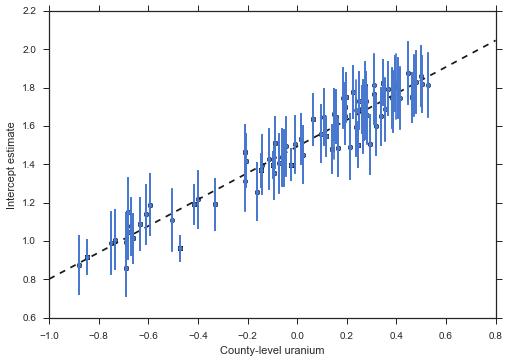The standard errors on the intercepts are narrower than for the partial-pooling model without a county-level covariate.

### Correlations among levels¶

In some instances, having predictors at multiple levels can reveal correlation between individual-level variables and group residuals. We can account for this by including the average of the individual predictors as a covariate in the model for the group intercept.

$$\alpha_j = \gamma_0 + \gamma_1 u_j + \gamma_2 \bar{x} + \zeta_j$$

These are broadly referred to as contextual effects.

In :
# Create new variable for mean of floor across counties
xbar = srrs_mn.groupby('county')['floor'].mean().rename(county_lookup).values

In :
x_mean = xbar[county]

In :
contextual_effect = """
data {
int<lower=0> J;
int<lower=0> N;
int<lower=1,upper=J> county[N];
vector[N] u;
vector[N] x;
vector[N] x_mean;
vector[N] y;
}
parameters {
vector[J] a;
vector b;
real mu_a;
real<lower=0,upper=100> sigma_a;
real<lower=0,upper=100> sigma_y;
}
transformed parameters {
vector[N] y_hat;

for (i in 1:N)
y_hat[i] <- a[county[i]] + u[i]*b + x[i]*b + x_mean[i]*b;
}
model {
mu_a ~ normal(0, 1);
a ~ normal(mu_a, sigma_a);
b ~ normal(0, 1);
y ~ normal(y_hat, sigma_y);
}
"""

In :
contextual_effect_data = {'N': len(log_radon),
'J': len(n_county),
'county': county+1, # Stan counts starting at 1
'u': u,
'x_mean': x_mean,
'x': floor_measure,

contextual_effect_fit = pystan.stan(model_code=contextual_effect, data=contextual_effect_data,
iter=1000, chains=2)

In :
contextual_effect_fit['b'].mean(0)

Out:
array([ 0.68837427, -0.68702399,  0.4069351 ])
In :
contextual_effect_fit.plot('b');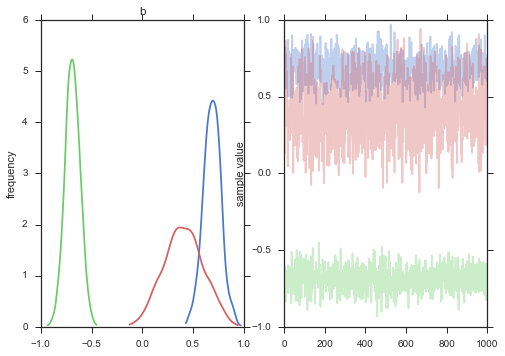So, we might infer from this that counties with higher proportions of houses without basements tend to have higher baseline levels of radon. Perhaps this is related to the soil type, which in turn might influence what type of structures are built.

### Prediction¶

Gelman (2006) used cross-validation tests to check the prediction error of the unpooled, pooled, and partially-pooled models

root mean squared cross-validation prediction errors:

• unpooled = 0.86
• pooled = 0.84
• multilevel = 0.79

There are two types of prediction that can be made in a multilevel model:

1. a new individual within an existing group
2. a new individual within a new group

For example, if we wanted to make a prediction for a new house with no basement in St. Louis county, we just need to sample from the radon model with the appropriate intercept.

In :
county_lookup['ST LOUIS']

Out:
69

That is,

$$\tilde{y}_i \sim N(\alpha_{69} + \beta (x_i=1), \sigma_y^2)$$

This is simply a matter of adding a single additional line in PyStan:

In :
contextual_pred = """
data {
int<lower=0> J;
int<lower=0> N;
int<lower=0,upper=J> stl;
real u_stl;
real xbar_stl;
int<lower=1,upper=J> county[N];
vector[N] u;